×#### Thank you for registering.

One of our academic counsellors will contact you within 1 working day.

Click to Chat

1800-1023-196

+91-120-4616500

CART 0

• 0

MY CART (5)

Use Coupon: CART20 and get 20% off on all online Study Material

ITEM
DETAILS
MRP
DISCOUNT
FINAL PRICE
Total Price: Rs.

There are no items in this cart.
Continue Shopping```Calculations Involving Unit Cells Dimensions

Calculations Involving Unit Cells Dimensions

Density of Unit Cell

Derived Results to Evaluate Density of Unit Cell

Simple or Primitive Unit Cell

Body-centred Unit Cell

Face-centred Unit Cell

Calculations Involving Unit Cells Dimensions

With the help of geometry and attributes of unit cells, we can easily evaluate the volume of the unit cell. With volume and mass of atoms, we can also evaluate the density of the unit cell. A crystal lattice is represented in terms of unit cells; we can determine the density of crystal lattices by evaluating the density of unit cells.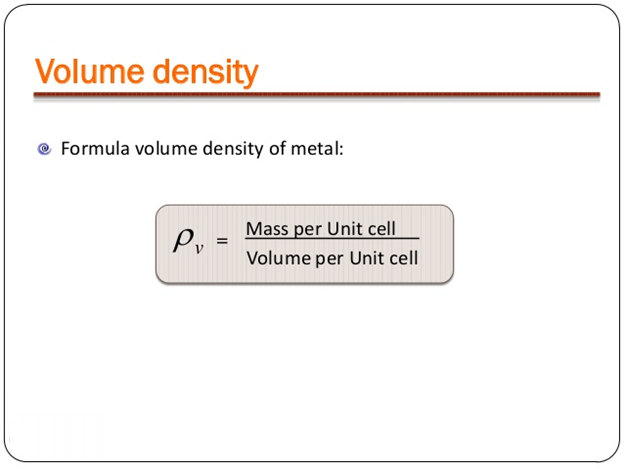Image 1: Volume of Unit Cell

Density of Unit Cell

A unit cell is a three-dimensional structure occupying one, two or more atoms. With the help of dimensions of unit cells, we can evaluate the density of the unit cell. To do so let’s consider a unit cell of edge length ‘a’, therefore the volume of the cell will be ‘a3’. Also, density is defined as the ratio of the mass of unit cell and volume of the unit cell.

So we can writeMass of unit cell varies with number of atoms “n” and mass of a single atom “m”. Mathematically mass of unit cell is the product of number of atoms “n” and mass of one atom “m” i.e.

Mass of Unit Cell = n × m

Also from quantitative aspect of atoms, mass of one atom can be written in terms of Avogadro Number ( NA ) and molar mass of atom ( M), that is,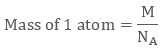Volume of Unit Cell = a3

Placing the required values in equation 1 we getTherefore if we know molar mass of atom “M”, number of atoms “n”, the edge length of unit cell “a” we can evaluate the density of unit cell.

Derived Results to Evaluate Density of Unit Cell

Following results can be used to derive density of unit cell:

Simple or Primitive Unit Cell

Body-centred Unit Cell

Face-centred Unit Cell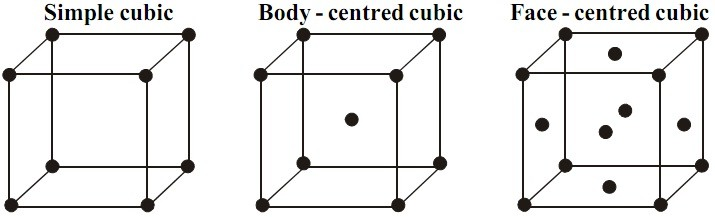Image 2: By knowing number of atoms we can easily determine volume of any type of unit cell

Simple or Primitive Unit Cell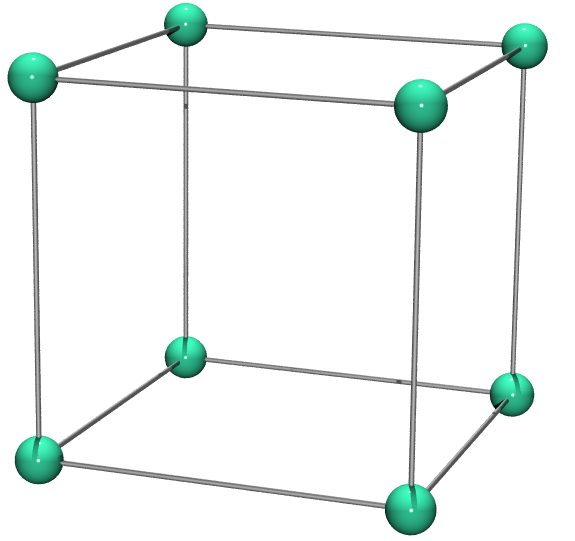Image 3: Simple Unit Cell

There is only one atom present in the simple or primitive unit cell. Therefore density of simple unit cell is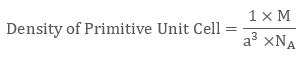Body-centred Unit CellImage 4: Body-centred Unit Cell

The value of number of atoms ‘n’ is 2, so density of body-centred unit cell isFace-centred Unit Cell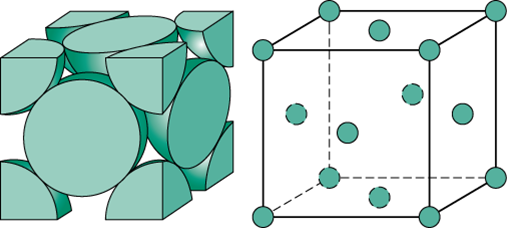Image 5: Face-centred Unit Cell

Face-centred Unit Cell contains 4 atoms, therefore its density is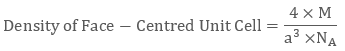Example 1

The density of a face-centred unit cell is 6.23 g cm-3. Given the atomic mass of a single atom is 60, evaluate the edge length of the unit cell. (Take value of NA = 6.022 × 1023)

As the unit cell is a face-centred unit cell, a number of atoms will be  4. We have

n = 4 , M = 60 , NA = 6.022 × 1023 and d = 6.23 g cm-3

We know that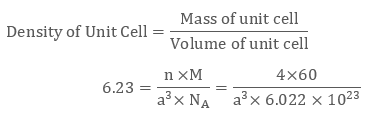After evaluating we get the value of edge length of the cube to be 4 × 10-8 cm.

Example 2

KBr or potassium bromide has density 2.75 g cm-3. The edge length of its unit cell is 654 pm. Prove that KBr depicts face-centred cubic structure.

We have edge length of unit cell = 654 pm = 6.54 × 10-8 cm

Therefore volume of the cell = (6.54 ×10-8)3 cm3

Molar Mass of Potassium Bromide ( KBr) is 119 g/mol

Density of KBr = 2.75 g cm-3

We know that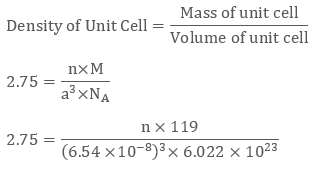Evaluating we get value of n to be 3.09 ≈ 4

Since a number of atoms is 4 we can clearly say that KBr is a face-centred cubic structure.

Watch this Video for more reference

Calculations Involving Unit Cells Dimensions
```### Course Features

• 731 Video Lectures
• Revision Notes
• Previous Year Papers
• Mind Map
• Study Planner
• NCERT Solutions
• Discussion Forum
• Test paper with Video Solution